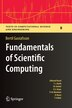# Fundamentals of Scientific Computing

## byBertil Gustafsson

### Pricing and Purchase Info

\$81.74 online
\$96.95 list price save 15%
Earn 409 plum® points

Prices and offers may vary in store

Quantity:

In stock online

Ships free on orders over \$25

Not available in stores

### about

The book of nature is written in the language of mathematics--Galileo Galilei

How is it possible to predict weather patterns for tomorrow, with access solely to today's weather data? And how is it possible to predict the aerodynamic behavior of an aircraft that has yet to be built?

The answer is computer simulations based on mathematical models - sets of equations - that describe the underlying physical properties. However, these equations are usually much too complicated to solve, either by the smartest mathematician or the largest supercomputer. This problem is overcome by constructing an approximation: a numerical model with a simpler structure can be translated into a program that tells the computer how to carry out the simulation.

This book conveys the fundamentals of mathematical models, numerical methods and algorithms. Opening with a tutorial on mathematical models and analysis, it proceeds to introduce the most important classes of numerical methods, with finite element, finite difference and spectral methods as central tools. The concluding section describes applications in physics and engineering, including wave propagation, heat conduction and fluid dynamics. Also covered are the principles of computers and programming, including MATLAB®.

Top CSE scientist
Loading
Title:Fundamentals of Scientific ComputingFormat:PaperbackDimensions:326 pagesPublished:August 3, 2013Publisher:Springer-Verlag/Sci-Tech/TradeLanguage:English

The following ISBNs are associated with this title:

ISBN - 10:3642268641

ISBN - 13:9783642268649

## Reviews

Table of Contents

Part I Models and elementary mathematics.- 1 Introduction.- 2 Mathematical models.- 3 Basic linear algebra.- 4 Analysis tools- 5 Elementary functions.- Part II Fundamentals in numerical analysis.- 6 The Fourier transform.- 7 Polynomial expansions.- 8 Least square problems.- Part III Numerical methods for differential equations.- 9 Numerical methods for difference methods.- 10 Finite difference methods.- 11 Finite element method.- 12 Spectral methods.- Part IV Numerical methods for algebraic equations.- 13 Numerical solutions of nonlinear equations.- 14 Linear systems of equations.- Part V Applications.- 15 Wave propagation.- 16 Heat conduction.- 17 Fluid dynamics.- 18 Computers and programming.- 19 Further reading.- A. Mathematical Rules.- References.- Index